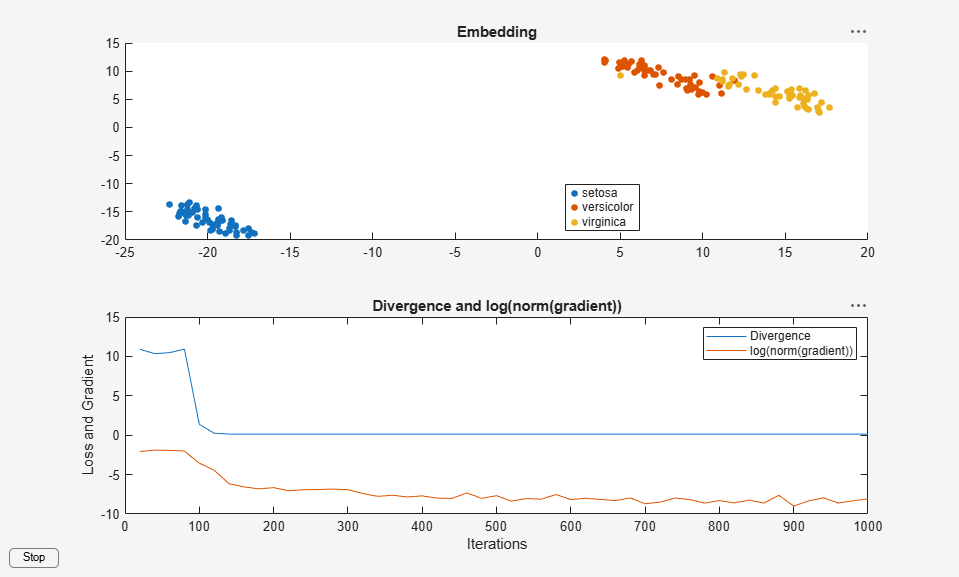## t-SNE Output Function

### t-SNE Output Function Description

A `tsne` output function is a function that runs after every `NumPrint` optimization iterations of the t-SNE algorithm. An output function can create plots, or log data to a file or to a workspace variable. The function cannot change the progress of the algorithm, but can halt the iterations.

Set output functions using the `Options` name-value pair argument to the `tsne` function. Set `Options` to a structure created using `statset` or `struct`. Set the `'OutputFcn'` field of the `Options` structure to a function handle or cell array of function handles.

For example, to set an output function named `outfun.m`, use the following commands.

```opts = statset('OutputFcn',@outfun); Y = tsne(X,'Options',opts);```

Write an output function using the following syntax.

```function stop = outfun(optimValues,state) stop = false; % do not stop by default switch state case 'init' % Set up plots or open files case 'iter' % Draw plots or update variables case 'done' % Clean up plots or files end```

`tsne` passes the `state` and `optimValues` variables to your function. `state` takes on the values `'init'`, `'iter'`, or `'done'` as shown in the code snippet.

### `tsne optimValues` Structure

optimValues FieldDescription
`'iteration'`Iteration number
`'fval'`Kullback-Leibler divergence, modified by exaggeration during the first 99 iterations
`'grad'`Gradient of the Kullback-Leibler divergence, modified by exaggeration during the first 99 iterations
`'Exaggeration'`Value of the exaggeration parameter in use in the current iteration
`'Y'`Current embedding

### t-SNE Custom Output Function

This example shows how to use an output function in `tsne`.

Custom Output Function

The following code is an output function that performs these tasks:

• Keep a history of the Kullback-Leibler divergence and the norm of its gradient in a workspace variable.

• Plot the solution and the history as the iterations proceed.

• Display a `Stop` button on the plot to stop the iterations early without losing any information.

The output function has an extra input variable, `species`, that enables its plots to show the correct classification of the data. For information on including extra parameters such as `species` in a function, see Parameterizing Functions (MATLAB).

```function stop = KLLogging(optimValues,state,species) persistent h kllog iters stopnow switch state case 'init' stopnow = false; kllog = []; iters = []; h = figure; c = uicontrol('Style','pushbutton','String','Stop','Position', ... [10 10 50 20],'Callback',@stopme); case 'iter' kllog = [kllog; optimValues.fval,log(norm(optimValues.grad))]; assignin('base','history',kllog) iters = [iters; optimValues.iteration]; if length(iters) > 1 figure(h) subplot(2,1,2) plot(iters,kllog); xlabel('Iterations') ylabel('Loss and Gradient') legend('Divergence','log(norm(gradient))') title('Divergence and log(norm(gradient))') subplot(2,1,1) gscatter(optimValues.Y(:,1),optimValues.Y(:,2),species) title('Embedding') drawnow end case 'done' % Nothing here end stop = stopnow; function stopme(~,~) stopnow = true; end end ```

Use the Custom Output Function

Plot the Fisher iris data, a 4-D data set, in two dimensions using `tsne`. There is a drop in the Divergence value at iteration 100 because the divergence is scaled by the exaggeration value for earlier iterations. The embedding remains largely unchanged for the last several hundred iterations, so you can save time by clicking the `Stop` button during the iterations.

```load fisheriris rng default % for reproducibility opts = statset('OutputFcn',@(optimValues,state) KLLogging(optimValues,state,species)); Y = tsne(meas,'Options',opts,'Algorithm','exact'); ```